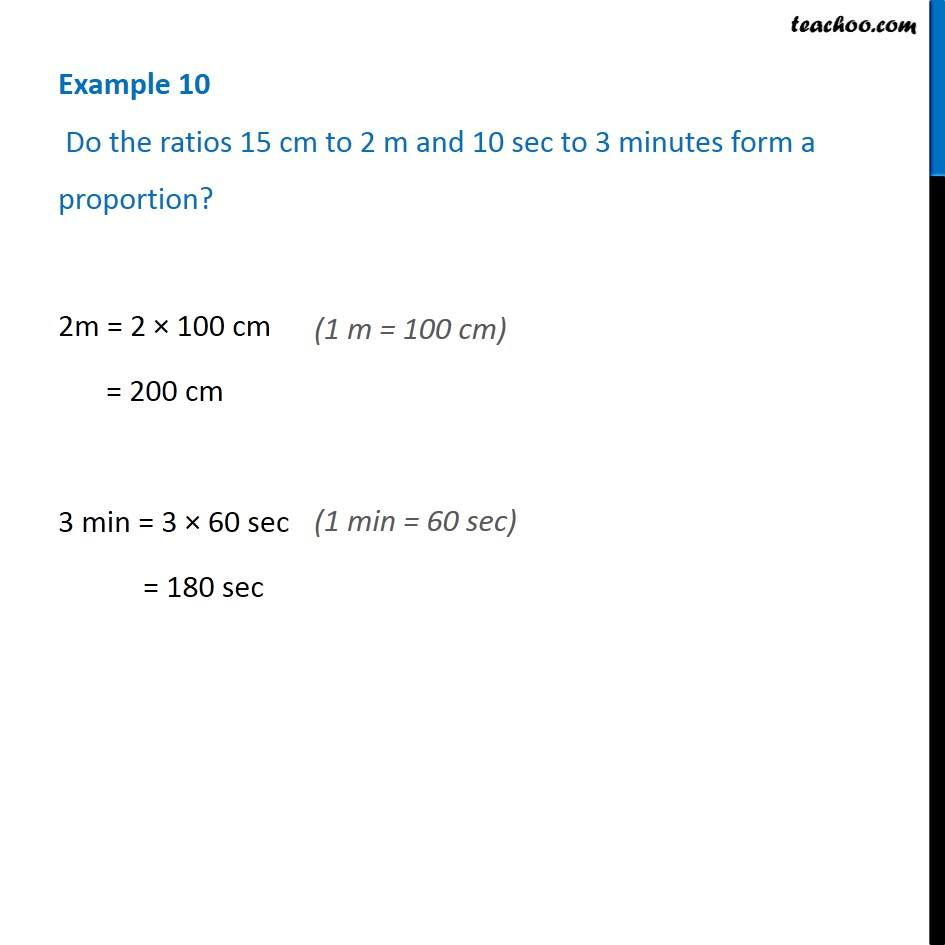Examples

Chapter 12 Class 6 Ratio and Proportion
Serial order wiseLearn in your speed, with individual attention - Teachoo Maths 1-on-1 Class

### Transcript

Example 10 Do the ratios 15 cm to 2 m and 10 sec to 3 minutes form a proportion?2m = 2 × 100 cm = 200 cm 3 min = 3 × 60 sec = 180 sec 15 cm : 2 m = (15 𝑐𝑚)/(2 𝑚) = (15 𝑐𝑚)/(200 𝑐𝑚) = 15/200 = 3/40 = 3 : 40 10 sec : 3 min = (10 𝑐𝑚)/(3 𝑚𝑖𝑛) = (10 𝑠𝑐)/(180 𝑠𝑒𝑐) = 10/180 = 1/18 = 1 : 18 ∴ 15 cm : 2 m ≠ 10 sec : 3 min Thus, Given ratios are not in proportion